Open in App
Not now

# Difference between “INNER JOIN” and “OUTER JOIN”

• Difficulty Level : Medium
• Last Updated : 10 Jun, 2021

JOINS :
Joins in SQL are used to combine rows from multiple tables on a specific condition, which is a relation between the columns of two tables. And there are different types of joins and in this article let us cover INNER JOIN and OUTER JOIN  and their differences.

Let us consider the two tables student and location and see how the differences would look like by combing tables using different joins.
Table1 –

student

Table2 –

location

Firstly, creating tables and inserting data into tables using MSSQL as a server:

• Creating the student tables and location using the following queries –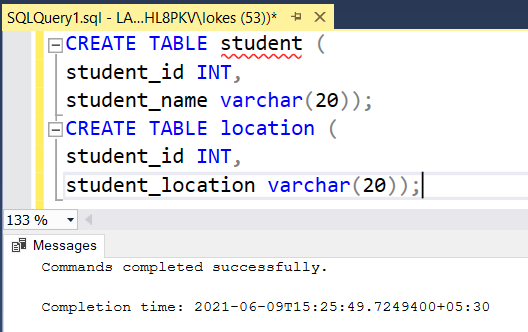Created two tables

• Inserting rows into student tables and location using the following queries –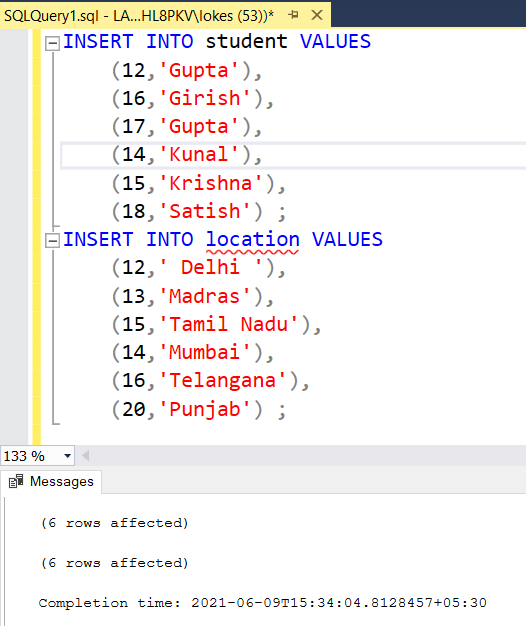Inserted data into tables

• Viewing the tables using the following query –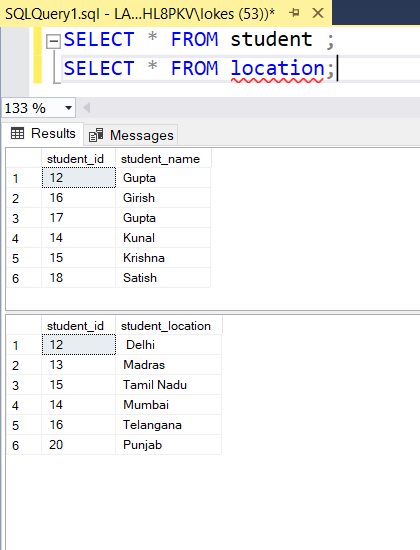Viewing data from tables

Types of JOINS :

1. INNER JOIN

• EQUI JOIN
• SELF JOIN

2. OUTER JOIN

• LEFT JOIN
• RIGHT JOIN
• FULL JOIN

1. Inner Join :
When the inner join is used, it considers only those attributes that we want to match both the table and, if anything that doesn’t, wouldn’t be included in our result table.
Two types of Inner Join –

• Equi Join –
It is the subcategory of Inner Join where it is restricted to only equality condition in the table. The join is said to be Equi join if and only if there is an equality condition in the query.
The query for Equi Join on the above two tables:
```SELECT * FROM
student
INNER JOIN
location
ON
student.student_id = location.student_id;```

OUTPUT TABLE –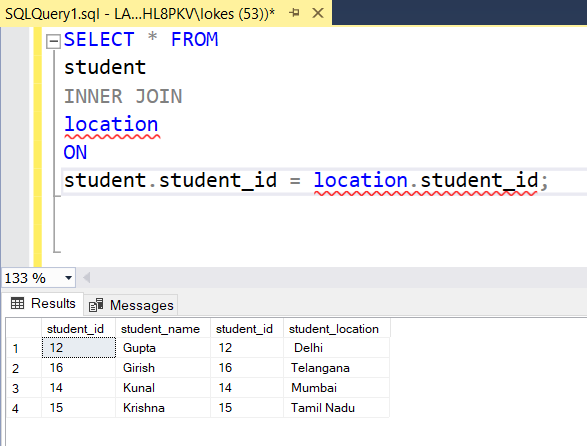• Self Join –
Self Join considers the same table as another table and outputs the resultant table after the required condition satisfies.

The query for Self Join is in the above two tables:

```SELECT s1.student_id ,s1.student_name FROM
student s1
INNER JOIN
student s2
ON
s1.student_name= s2.student_name AND s1.student_id<> s2.student_id;```

OUTPUT TABLE –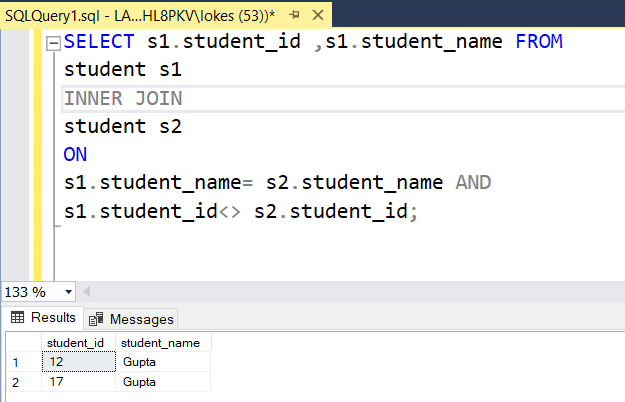Venn diagram representation of the Inner Join –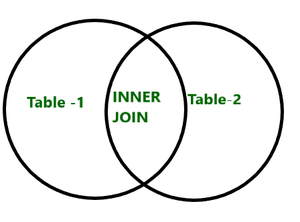2. Outer Join :
In the outer join, we consider any of the tables completely or both such that the remaining fields that were unmatched in both the tables were kept NULL.

Three types of Outer Join –
1. Left Join or (left outer join) –
In left join, we consider the left table completely and the matched attributes (based on condition) in the right table along with, the unmatched attributes of the left table with the right table are placed NULL with respect to the column in the left table.
The query for Left Join is in the above two tables:

```SELECT * FROM
student
LEFT JOIN
location
ON
student.student_id = location.student_id;```

OUTPUT TABLE: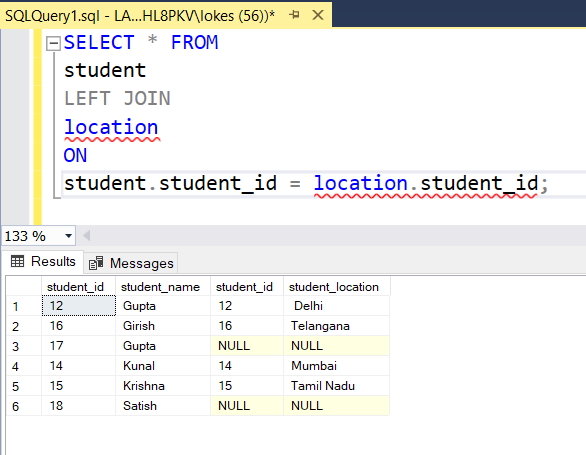Venn diagram representation of the Left Join: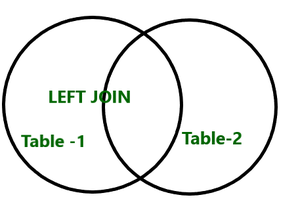Right, Join –
In the right join, we consider the right table completely and the matched attributes (based on condition) in the left table along with, the unmatched attributes of the right table with the left table are placed NULL with respect to a column in the right table.
The query for Right Join is in the above two tables:

```SELECT * FROM
student
RIGHT JOIN
location
ON
student.student_id = location.student_id;```

OUTPUT TABLE –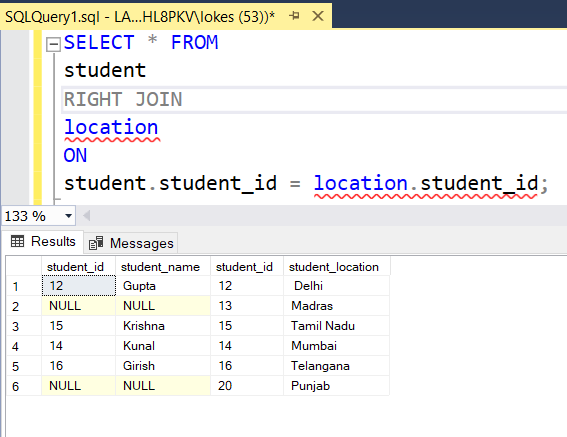Venn diagram representation of the Right Join –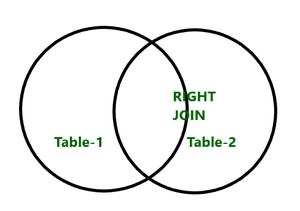Full Join –
It is the union of both left join and right join where all the columns of the left table and the right table are considered where the unmatched or unfound attributes of the left table or right table will be placed with NULL in the resultant table.
The query for Full Join is in the above two tables:

```SELECT * FROM
student
FULL JOIN
location
ON
student.student_id = location.student_id;```

OUTPUT TABLE –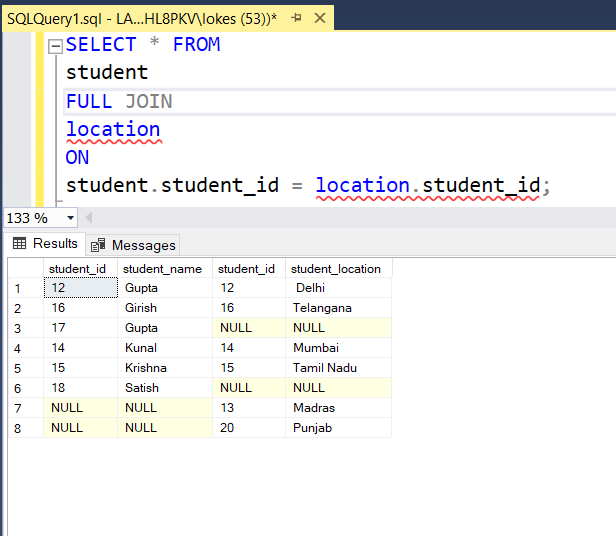Venn diagram representation of the full Join –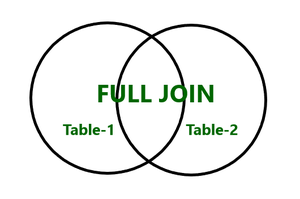My Personal Notes arrow_drop_up
Related Articles